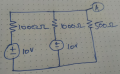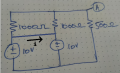# Calculating the voltage at Node A

#### Electronic_Maniac

Joined Oct 26, 2017
253
Hi all,

I need to calculate the voltage at node A at the below circuit. Please tell me whether my approach is rightSo, I am assuming that the 1000ohms in series with the voltage sources as internal resistances.

Current through the 500ohm would be, I = 10/500 = 20mA

But we need to consider the internal resistance of 1000ohms right
So, terminal voltage, Er = V - IR, Er = 10 - (20mA)*(1000Ohms) = 10-20 = -10V ?

What am i doing wrong here?

#### Bordodynov

Joined May 20, 2015
2,745
For me, the answer is obvious - +5V.
Connect the upper pins of the voltage sources and discard one of the sources. The resistance of the two parallel 1kOhm resistors is 500ohms. The result is a simple, resistive divider.
And whoa-la - =5V

•OBW0549

#### Electronic_Maniac

Joined Oct 26, 2017
253
For me, the answer is obvious - +5V.
Connect the upper pins of the voltage sources and discard one of the sources. The resistance of the two parallel 1kOhm resistors is 500ohms. The result is a simple, resistive divider.
And whoa-la - =5V
I couldn't understand you. Could you please explain more briefly

#### DickCappels

Joined Aug 21, 2008
6,819
I see one place you went wrong:
" Current through the 500ohm would be, I = 10/500 = 20mA"

Clearly there are resistors in series with the 10 volt batteries before the 500 ohm resistor so you cannot possibly have 10V across the 500 ohm resistor.

Can you write formulas that express the current through the branches of the circuit?

#### WBahn

Joined Mar 31, 2012
26,398
Hi all,

I need to calculate the voltage at node A at the below circuit. Please tell me whether my approach is right

View attachment 190436

So, I am assuming that the 1000ohms in series with the voltage sources as internal resistances.

Current through the 500ohm would be, I = 10/500 = 20mA

But we need to consider the internal resistance of 1000ohms right
So, terminal voltage, Er = V - IR, Er = 10 - (20mA)*(1000Ohms) = 10-20 = -10V ?

What am i doing wrong here?

Why are you using 10 V as the voltage across the 500 Ω resistor?

It seems you are making the all-too-common mistake of throwing whatever voltage happens to be handy at Ohm's Law and since 10 V is the only voltage in the diagram, that's what you used. But Ohm's Law requires that you use the voltage across the resistor in question and there is no reason to expect that to be 10 V.

With some experience, you can use Bordodynov's trick, but it is a bit much to expect you to spot it at the point in your education that you are at.

Speaking of the point where you are at, we need a bit more detail about that in order to help you work the problem using the techniques you are currently learning. Have you been exposed to mesh current analysis or node voltage analysis yet? My guess is no. How about KVL and KCL? My guess is that this is about where you are at. If so, define the node voltages and branch currents on your diagram and then write the loop equations and KCL equations and let's make sure you've got them correct before proceeding.

#### Electronic_Maniac

Joined Oct 26, 2017
253
I am still not able to understand when you have two voltage sources having the same value. I understand and can try using KCL and KVL. I just want to understand intuitively. How can me remove one source as mentioned in #2

#### MrChips

Joined Oct 2, 2009
22,552
In this example, since the voltage sources and series resistors are identical, you can connect the components in parallel and use the parallel equivalent as in post #2.

If any voltage source or resistance is different, you cannot make this simplification. You would have to use KVL and KCL to solve.

#### WBahn

Joined Mar 31, 2012
26,398
I am still not able to understand when you have two voltage sources having the same value. I understand and can try using KCL and KVL. I just want to understand intuitively. How can me remove one source as mentioned in #2
Imagine connecting a wire between the two sources as shown below:What is the current i?

By symmetry the current has to be zero since the relevant part of the circuit on the left side of the wire is the same as on the right, so the current flowing left-to-right must be the same as the current flowing right-to-left and the only way that is possible is if i=0.

Since i=0, adding the wire has no effect on any of the other voltages and currents in the circuit.

But now we can clearly see that the 10 V sources are in parallel with each other and the 1000 Ω resistors are in parallel with each other, so both can be reduced to their parallel equivalents.

•Electronic_Maniac

#### Yeye

Joined Nov 12, 2019
47
It is quite simple. You can imagine the 2 voltage sources to be only 1. Then, the current flowing through one of the Voltage source will be doubled, but that's it, nothing else will change.
It should be clear then, the 1k Ohm Resistors are in parallel, so they equal one 500 Ohm resistor. This 500 ohm resistor is in series with the other 500 Ohm one. 2 equal series resistors build a 50% Voltage divider. The voltage at the node is 5V ( 10v / 2 = 5v )

Or a bit more complicated: The calculated 500 Ohm resistor and the given 500 ohm one equal 1k Ohm together in series. At 10V, there are 10mA flowing. 500 Ohm*0,010 A= 5V.

The 10m A flowing just split between both the power supplies, since they are equal voltage and in parallel to each other.